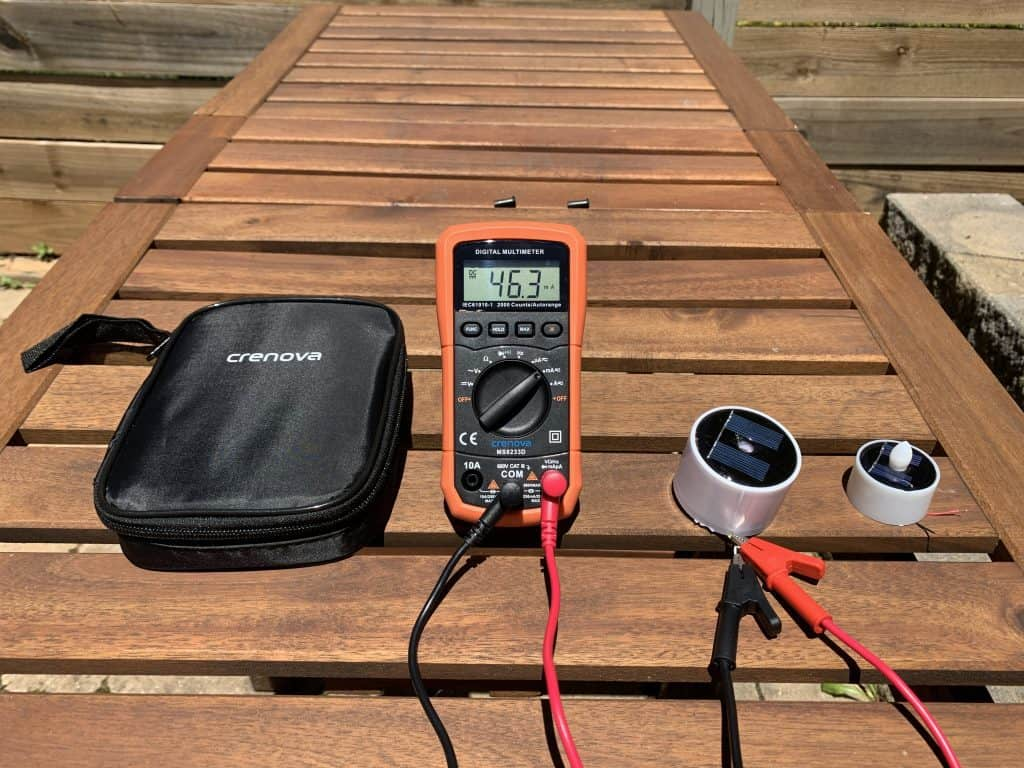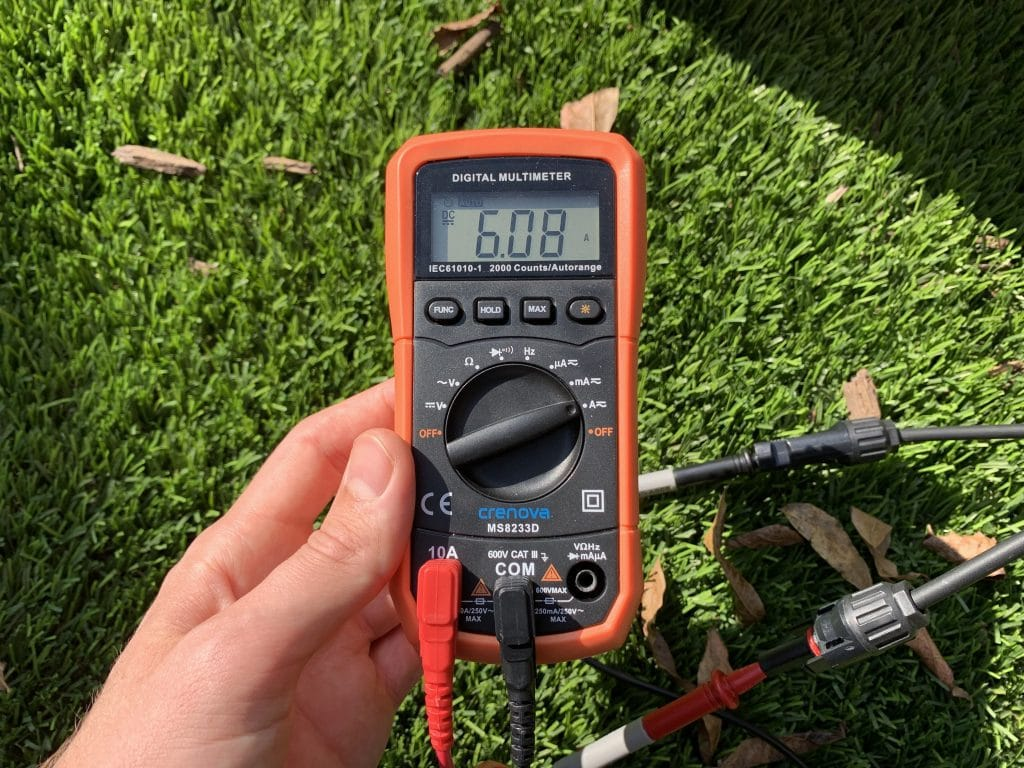# Milliamps to Amps (mA to A) Conversion Calculator

Use our interactive calculator to easily convert milliamps to amps (mA to A).

Error: Enter a valid number of milliamps.
amps
Conversion formula: A = mA ÷ 1000

Or would you rather convert amps to milliamps?

## Milliamps to Amps Conversion Chart

Here's a chart converting common milliamp values to amps.

## How to Convert Milliamps to Amps (mA to A)

To convert milliamps to amps, divide the number of milliamps by 1000.

Formula: amps = milliamps ÷ 1000

Abbreviated: A = mA ÷ 1000

### Example

For example, I tested the solar panel output from some small solar tea candles. The panel on one of the candles had a short circuit current of 46.3 milliamps.When I tested the tiny solar panel on this solar candle, I measured a short circuit current of 46.3 milliamps, or 0.0463 amps.

Here's how to convert this number to amps:

`46.3 mA ÷ 1000 = 0.0463 A`

So, in this example, it would also be correct to say the solar panel has a short circuit current of 0.0463 amps.

That's all there is to it. 🙂

## How to Convert Amps to Milliamps (A to mA)

To convert the other way, multiply amps times 1000.

Formula: milliamps = amps × 1000

Abbreviated: mA = A × 1000

### Example

To stick with the theme of solar panels, let's say you measure the short circuit current of a large 100 watt solar panel. You get a short circuit current of 6.08 amps.This 100 watt solar panel has a short circuit current of 6.08 amps, or 6080 milliamps.

To convert this value to milliamps, you do the following:

`6.08 A × 1000 = 6080 mA`

So, expressed in milliamps, you would say this panel has a short circuit current of 6080 milliamps.

## More Electricity Calculators